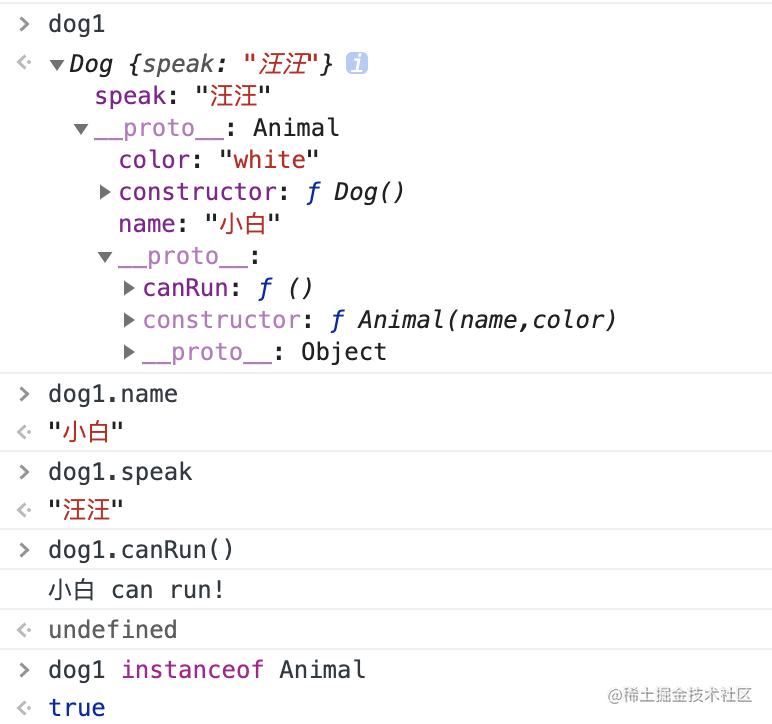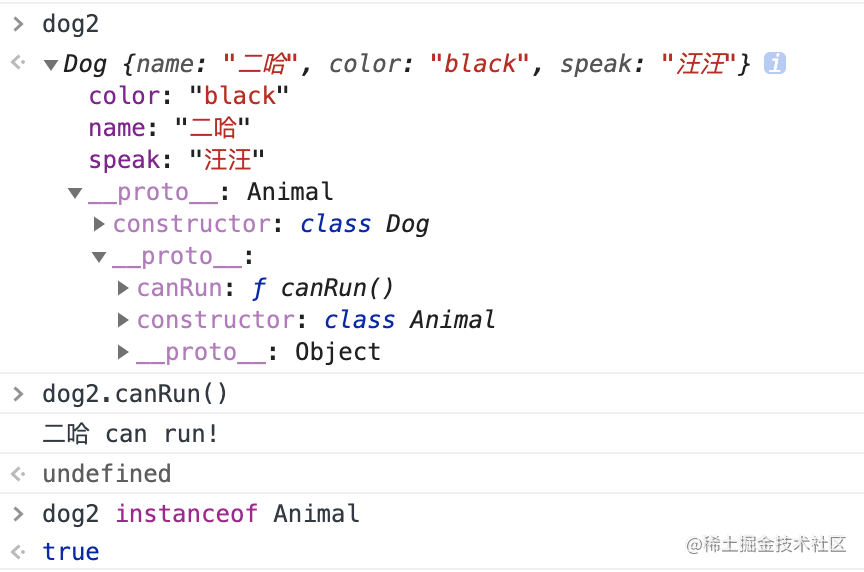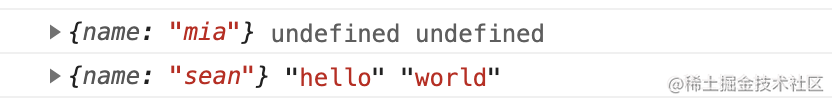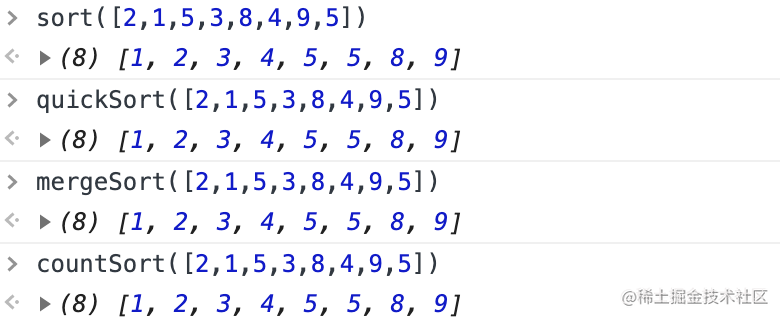# 「一」你如何理解JS的继承？

## 1. 基于 原型 的继承

• 子类的原型指向父类的实例对象来实现父类属性和方法的继承；
• 因为父类实例对象的构造函数constructor指向了父类原型，所以需要将子类原型的构造函数constructor指向子类构造函数

### 代码举例

``````function Animal(name,color){
this.name = name
this.color = color
}
Animal.prototype.canRun = function(){
console.log(`\${this.name} can run!`)
}
function Dog(){
this.speak = '汪汪'
}
Dog.prototype = new Animal('小白','white')
Dog.prototype.constructor = Dog

let dog1 = new Dog()## 2. 基于 class 的继承

class实现继承的核心在于使用`extends`表明继承自哪个父类，并且在子类构造函数中必须调用`super`，它可以被看成`Animal.call(this, name, color)`

### 代码举例

``````class Animal{
constructor(name,color) {
this.name = name
this.color = color
}
canRun(){
console.log(`\${this.name} can run!`)
}
}
class Dog extends Animal{
constructor(name,color,speak) {
super(name,color)
this.speak = '汪汪'
}
}
let dog2 = new Dog('二哈','black')# 「二」闭包

## 1. 什么是闭包

### 代码举例

``````function f1(){
let a1=1;
function f2(){
console.log(a1)
}
return f2
}
let result=f1()
result();//1

## 2. 闭包的用途

• 通过闭包，外部环境可以读取函数内部的变量。

``````function f1(){
let a1=1;
function f2(){
return a1;
}
return f2
}
let result=f1()
result();

• 让这些变量的值始终存在内存中。不会在f1调用后被自动清除。
• 使用闭包，内部函数变量不会污染外部变量，规避冲突

## 3. 闭包的缺点

• 局部变量会常驻内存，造成内存溢出（有一块内存空间被长期占有而不被释放），解决方法是，在退出函数之前，将不使用的局部变量全部删除。

### 面试题

let result=f1()将，f2函数赋值给了result，f2函数被标记了一次引用，执行完result()之后，f2还存在内存中，包括f2中的闭包变量a1，所以多次调用result()，时打印的a1++的值是从闭包变量这个作用域中取得的。这个值又常驻内存，所以会a1的值会变化。

# 「三」call、apply、bind 的用法

this 永远指向最后调用它的那个对象。
call、apply、bind这三个函数的作用是改变一个函数在执行时this的指向。

## 1. call 的用法

`fn.call(xxx, 1, 2, 3)`

``````Array.prototype.forEach2 = function(fn) {
for (let i = 0; i < this.length; i++) {
fn(this[i], i)
}
}

let arr = [1,2,3]
arr.forEach2.call(arr, (item)=>console.log(item))

## 2. apply 的用法

`fn.call(xxx, [1, 2, 3])`

### 求数组里的的最大值和最小值

``````const array = [15, 6, 24, 3, 9]
const max = Math.max.apply(null, array)
const min = Math.min.apply(null, array)

console.log(max)  // 24
console.log(min)  //  3

## 3. bind 的用法

``````function f1(p1,p2) {
console.log(this, p1, p2)
}
let f2 = f1.bind({name: 'mia'})
f2()
let f3 = f1.bind({name: 'sean'}, 'hello', 'world')
f3()# 「四」面试题：如何实现数组去重

## 1. 不使用 Set 实现

### 方法一 (indexOf())

• 新建一个空数组
• 遍历原数组
• 用indexOf() 判断循环出来的元素在新数组中是否存在，不存在则把数据推送至新数组里面
``````function unique(array){
let result = []
for(let i=0; i<array.length; i++){
if(result.indexOf(array[i]) === -1){
result.push(array[i])
}
}
return result
}

unique([1,5,2,3,4,2,3,1,3,4])  // [1,5,2,3,4]

### 方法二 (indexOf() / splice())

• 遍历数组
• 用indexOf() 判断循环出来元素下标是否和元素在数组中的索引是否不相等
• 如果不相等（则说明这是一个重复的元素），则把该元素从数组中删除
• 删除后数组长度变短，所以索引也要减一
``````function unique(array){
for(let i=0; i<array.length; i++){
if(array.indexOf(array[i]) != i){
array.splice(i, 1)
i--       // 因为每次遇到相同的元素删除后，数组的长度会改变，所以数组的下标也要减一
}
}
return array
}

unique([1,5,2,3,4,2,3,1,3,4])   // [1,5,2,3,4]

### 方法三 (reduce() / includes())

• reduce()初始化一个空数组
• 用includes() 判断新数组是否包含原数组的元素
• 如果没有，则把数据推送至新数组里面
``````function unique(array){
return array.reduce((result,item)=>{
if(!result.includes(item)) {
result.push(item)
}
return result
},[])
}

unique([1,5,2,3,4,2,3,1,3,4]) // [1,5,2,3,4]

## 2. 使用 Set

### 方法一 (new Set() / Array.from())

• new Set() 去重
• Array.from()转成数组
``````function unique(array){
let result = new Set(array)
return Array.from(result)
}

unique([1,5,2,3,4,2,3,1,3,4]) // [1,5,2,3,4]

### 方法二 (new Set() / ... )

``````function unique(array) {
return [...new Set(array)]
}

unique([1,5,2,3,4,2,3,1,3,4])  // [1,5,2,3,4]

set去重注意 (数组中包含引用类型数据)
ES6中set可以实现数组去重，但是如果数组中的数据类型为引用类型时，是无法实现去重的。不支持对象数组和NaN。

## 3. 使用了 Map / WeakMap 以支持对象去重的

### 方法 (new Map())

``````function unique(array) {
let map = new Map();
let result = [];
for (let i=0; i < array.length; i++) {
if(map.has(array[i])) {
map.set(array[i], true);
} else {
map.set(array[i], false);
result.push(arr[i]);
}
}
return result;
}

unique([1,5,2,3,4,2,3,1,3,4])  // [1,5,2,3,4]

# 「五」数组排序

### 1. 选择排序

``````let minIndex = (numbers) => {
let index = 0
for (let i = 1; i < numbers.length; i++) {
if (numbers[i] < numbers[index]) {
index = i
}
}
return index
}

let swap = (array, i, j) => {
let temp = array[i]
array[i] = array[j]
array[j] = temp
}

let sort = (numbers) => {
for (let i = 0; i < numbers.length - 1; i++) {
let index = minIndex(numbers.slice(i)) + i
if (index !== i) {
swap(numbers, index, i)
}
}
return numbers
}

### 2. 快速排序

``````let quickSort = (arr) => {
if (arr.length <= 1) {
return arr
}
// 得到基准下标和基准数
let pivotIndex = Math.floor(arr.length / 2)
let pivot = arr.splice(pivotIndex, 1)
let left = []
let right = []
for (let i = 0; i < arr.length; i++) {
arr[i] < pivot ? left.push(arr[i]) : right.push(arr[i])
}
return quickSort(left).concat([pivot], quickSort(right))
}

### 3. 归并排序

``````let mergeSort = (arr) => {
// 精髓 如果长度是1 就默认排好序
if (arr.length === 1) {
return arr
}
let left = arr.slice(0, Math.floor(arr.length / 2))
let right = arr.slice(arr.length / 2)
return merge(mergeSort(left), mergeSort(right))
}

let merge = (a, b) => {
if (a.length === 0) {
return b
}
if (b.length === 0) {
return a
}
return a > b ?
[b].concat(merge(a, b.slice(1))) :
[a].concat(merge(a.slice(1), b))
}

### 4. 计数排序

``````let countSort = (arr) => {
let hashTable = {}, max = 0, result = []
for(let i=0; i<arr.length; i++){  // 遍历数组
!(arr[i] in hashTable) ?
hashTable[arr[i]] = 1 :
hashTable[arr[i]] += 1
if(arr[i] > max){ max=arr[i] }
}
for(let j=0; j<=max; j++){  // 遍历哈希表
if(j in hashTable){
for(let i=0; i<hashTable[j]; i++){
result.push(j)
}
}
}
return result
}470No more data